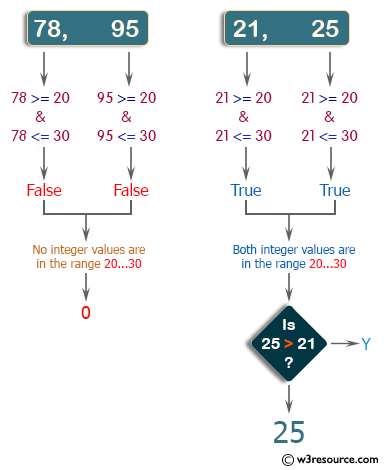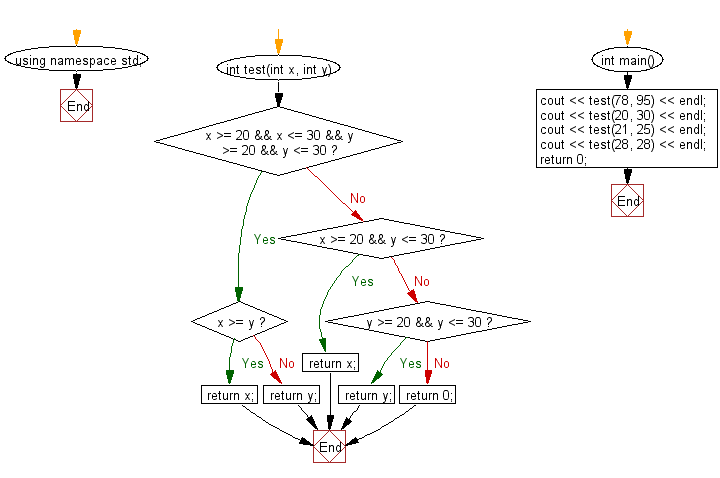﻿ C++ : Find the larger value from two positive integer values# C++ Exercises: Find the larger value from two positive integer values that is in the range 20..30 inclusive

## C++ Basic Algorithm: Exercise-21 with Solution

Write a C++ program to find the larger value from two positive integer values that is in the range 20..30 inclusive, or return 0 if neither is in that range.

Sample Solution:

C++ Code :

``````#include <iostream>

using namespace std;

int test(int x, int y)
{
if (x >= 20 && x <= 30 && y >= 20 && y <= 30)
{
if (x >= y)
{
return x;
}
else
{
return y;
}
}
else if (x >= 20 && y <= 30)
{
return x;
}
else if (y >= 20 && y <= 30)
{
return y;
}
else
{
return 0;
}
}

int main()
{
cout << test(78, 95) << endl;
cout << test(20, 30) << endl;
cout << test(21, 25) << endl;
cout << test(28, 28) << endl;
return 0;
}
``````

Sample Output:

```0
30
25
28
```

Pictorial Presentation:Flowchart:C++ Code Editor:

Contribute your code and comments through Disqus.

What is the difficulty level of this exercise?

﻿